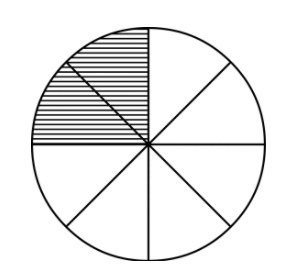# Decimal to Fraction Calculator

Simply input the decimal value and click calculate to find its fraction form using this calculator.## Decimal to fraction Calculator

This decimal to fraction calculator is used to convert the decimal values into proper or improper fractions. It will show you all the steps involved in the conversion process.

## What is a fraction?

A fraction is a part of the whole piece. It is the value that tells us what amount of part a thing is to the whole. E.g 2 pieces out of 8, 3 people from a family of 5 e.t.c.A fraction consists of two values; numerator and denominator. These values are separated by a slash (/). E.g ⅔, ½ e.t.c

Usually, fraction exits in decimal form. To easily use them in calculations, decimal to fraction conversion is performed.

## How to turn a decimal into a fraction?

For converting a decimal to a fraction, firstly, the decimal point is removed. This is done by multiplying the numerator and denominator with a multiple of 10.

After that GCF is used as a divisor. For further explanation, see this example.

### Example:

Convert the decimal value 1.2 to a fraction.

Solution:

Step 1: Write the decimal as a fraction.

= 1.2 / 1

Step 2: Since there is one digit after the decimal point, multiply by 10.

= 12 / 1 X 10 / 10 = 12 / 10

Step 3: Divide by GCF.

= 12 ÷ 2 / 10 ÷ 2 = 6 / 5

Hence 6/5 is the fraction form of 1.2.